StatLect

# Posterior odds ratio

The posterior odds ratio is the ratio between the posterior probabilities of two events.

In Bayesian inference, it is used to compare different hypotheses or different models.## Definition

Let,andbe three events.

We can use Bayes' rule to compute the conditional probabilities ofandgiven: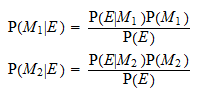The ratio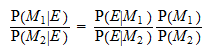is called the posterior odds ratio ofand.

The eventis often called evidence.

## Prior odds

The ratio of prior probabilitiesis called prior odds ratio.

## Bayes factor

Finally, the ratio of likelihoods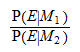is called Bayes factor.

Therefore,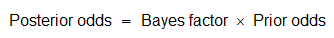## Interpretation

The interpretation of the posterior odds ratio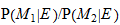is pretty simple:

• if the ratio is larger than 1, thenis more likely thanafter conditioning on the evidence;

• if the ratio is smaller than 1, thenis less likely thanconditional on.

Moreover, the ratio tells us exactly how much more likelyis than.

## Models

Typically, in Bayesian inference, the evidenceis some observed data, andandare two statistical models, that is, two sets of probability distributions that could have generated the data.

In other words, the posterior odds ratio betweenandquantifies how much more likelyis than, based on the prior and the evidence provided by the data.

Note that the two sets of probability distributions can be singletons, that is, the comparison can involve single probability distributions.

## The role of marginal likelihoods

Let us denote the data by(instead of) and probability density (or mass) functions by, as we did in previous lectures.

When there are two sets of parametric models, the priors are usually assigned in a hierarchical fashion.

In other words, we specify:

1. the probabilities of the two models,and;

2. two parametrized likelihoods,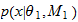and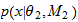;

3. two prior distributions over the parameters of the two models,and.

The posterior odds ratio is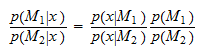The two quantitiesandare the so-called prior predictive distributions or marginal likelihoods.

If we are dealing with probability densities, the marginal likelihoods are obtained by computing two integrals: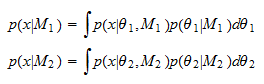This shows an important fact (see, e.g., O'Hagan 2006): in a comparison of two hierarchical models, the value of the Bayes factordepends not only on the evidence provided by the data, but also on the prior distributions of the parameters.

Therefore, a Bayes factor can incorporate both objective (the data) and subjective (the priors) information.

## What statisticians do

Although the posterior odds ratio can be used to neatly compare two models by using both prior information and the evidence provided by the data, statisticians often prefer to discuss and report Bayes factors.

Indeed, most of the books and papers that discuss Bayesian model comparisons (see, e.g., the marvellous introduction by Williams et al. 2017) focus almost exclusively on the properties of Bayes factors.

This is probably due to the fact that Bayes factors offer a more objective means of comparing models, by factoring out the more subjective prior odds ratio.

## References

O'Hagan, T. (2006) Bayes factors, Significance, 3, 184-186.

Williams, M. N., Baath, R. A. and Philipp, M. C. (2017). Using Bayes factors to test hypotheses in developmental research, Research in Human Development, 14, 321-337.